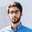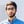# How to Compare Two Objects in JavascriptNouman Abbasi

Usually, when you compare data types like int and strings in JavaScript, you use the equality operators (== and ===). However, comparing objects with == and === will not work.

To fix this, one option is to stringify both objects and then use the equality operators.

### Using JSON.stringify()

We first convert the objects to their stringified version using JSON.stringify() and then compare them using ===:

const obj1 = {"a": 1, "b": 2};const obj2 = {"a": 1, "b": 2};console.log(JSON.stringify(obj1) === JSON.stringify(obj2)); // true
Using JSON.stringify()

If the order of the properties is different, the above method will evaluate as false even though the properties are the same:

const obj1 = {"a": 1, "b": 2};const obj2 = {"b": 2, "a": 1};console.log(JSON.stringify(obj1) === JSON.stringify(obj2)); // false
Using JSON.stringify() with different property order

To overcome this issue, we can use a JavaScript library, called lodash, instead.

### Using lodash

lodash is a JavaScript library that offers _.isEqual(value1, value2), which performs a deep comparison between two values to check if they are equivalent.

import _ from "lodash";const obj1 = {"a": 1, "b": 2};const obj2 = {"b": 2, "a": 1};console.log(_.isEqual(obj1, obj2)); // true
Using lodash

### Using ES6

Javascript(ES6) can also be used without using any third-party library to systematically compare the two objects. The order of the properties in the two objects is different.

const obj1 = { name: "Jack", age: 25 };const obj2 = { age: 25, name: "Jack" };let objEqual = false;const obj1Keys = Object.keys(obj1).sort();const obj2Keys = Object.keys(obj2).sort();if (obj1Keys.length !== obj2Keys.length) {  console.log(objEqual);} else {  const areEqual = obj1Keys.every((key, index) => {    const objValue1 = obj1[key];    const objValue2 = obj2[obj2Keys[index]];    return objValue1 === objValue2;  });  if (areEqual) {    objEqual = true;    console.log(objEqual);  } else {    console.log(objEqual);  }}
Using ES6

In the code above, we define two objects in lines 1–2 with the same key values pairs but in different order. After that, in lines 4–5, we sort both objects according to their keys.

In lines 6–20 we compare both objects with the help of the if statement. If both object properties are equal, it will evaluate to true else false.

RELATED TAGS

javascript
lodash

CONTRIBUTORNouman Abbasi